# 蜂窝陶瓷换热器的预热特性Preheating Characteristics of Honeycomb Ceramic Heat Exchanger

DOI: 10.12677/MOS.2021.101015, PDF, HTML, XML, 下载: 67  浏览: 126

Abstract: Aiming at the heat exchange process of high-temperature flue gas and air passing through the stacked honeycomb ceramic regenerator, based on the single-hole regenerator model, numerical simulations were carried out to study the effects of flow velocity and switching time on the tem-perature distribution of fluid and honeycomb ceramics during the preheating process, and the ef-fect on the temperature efficiency reached after the heat exchange was stabilized. The results show that under the conditions of high temperature flue gas inlet temperature 1123.15 K and air inlet temperature 313.15 K, when the switching time is 60 s, as the face velocity increases from 0.75 m/s to 1.5 m/s, the air outlet temperature at the end of heat release period changes from 1083.83 K to 1055.05 K, and the temperature efficiency drops from 95.15% to 91.59%, when the switching time is 90 s, as the face velocity increases from 0.75 m/s to 1.5 m/s, the air outlet temperature at the end of heat release period drops from 1076.25 K to 1040.37 K, and the temperature efficiency drops from 94.21% to 89.78%. Under the same face velocity, the shorter the switching time, the lower the flue gas outlet temperature during the heat storage period, and the higher the air outlet temperature and temperature efficiency during the heat release period. Under the same switching time, when the inlet flow rate increases, the flue gas outlet temperature during the heat storage period increases, and the air outlet temperature decreases during the heat release period as well as the temperature efficiency. The thermal inertia of the heat exchange process in the honeycomb ceramic regenerator is very strong, and it takes a long time to stabilize.

1. 引言

2. 模型简介

2.1. 物理模型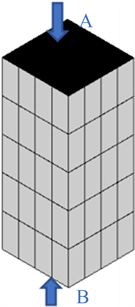Figure 1. Schematic diagram of accumulation state of regenerator

2.2. 网格划分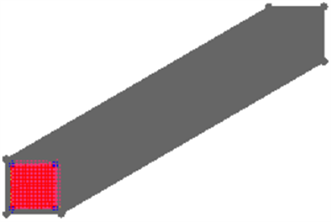Figure 2. Three dimensional single hole mesh model of stacked honeycombs

2.3. 数学模型

1) 高温烟气或空气流入蓄热体的温度和流速在入口界面上是均匀的；

2) 蓄热体的表面积及质量分布是均匀的；

3) 换向瞬间完成。

$\frac{\partial \rho }{\partial t}+\frac{\partial \left(\rho u\right)}{\partial x}+\frac{\partial \left(\rho v\right)}{\partial y}+\frac{\partial \left(\rho w\right)}{\partial z}=0$ (1)

$\frac{\partial \left(\rho u\right)}{\partial t}+div\left(\rho uu\right)=-\frac{\partial p}{\partial x}+\frac{\partial {\tau }_{xx}}{\partial x}+\frac{\partial {\tau }_{yx}}{\partial y}+\frac{\partial {\tau }_{zx}}{\partial z}+{F}_{x}$ (2)

$\frac{\partial \left(\rho v\right)}{\partial t}+div\left(\rho vu\right)=-\frac{\partial p}{\partial y}+\frac{\partial {\tau }_{xy}}{\partial x}+\frac{\partial {\tau }_{yy}}{\partial y}+\frac{\partial {\tau }_{zy}}{\partial z}+{F}_{y}$ (3)

$\frac{\partial \left(\rho v\right)}{\partial t}+div\left(\rho wu\right)=-\frac{\partial p}{\partial z}+\frac{\partial {\tau }_{xz}}{\partial x}+\frac{\partial {\tau }_{yz}}{\partial y}+\frac{\partial {\tau }_{zz}}{\partial z}+{F}_{z}$ (4)

$\frac{\partial \left(\rho T\right)}{\partial t}+div\left(\rho uT\right)=div\left(\frac{k}{{c}_{p}}grad|T\text{​}\text{​}\right)+{S}_{T}$ (5)

$\frac{\partial \left({\rho }_{s}{T}_{s}\right)}{\partial t}=div\left(\frac{{k}_{s}}{{c}_{p,s}}grad\text{​}\text{​}|{T}_{s}\right)+{S}_{T,s}$ (6)

2.4. 参数设置Table 1. Parameters of simulated working conditions

3. 预热过程中气体温度分布的演变Figure 3. Evolution of flue gas temperature distribution at the end of endothermic periodsFigure 4. Flue gas outlet temperature at the end of endothermic periodsFigure 5. Evolution of air temperature distribution at the end of exothermic periods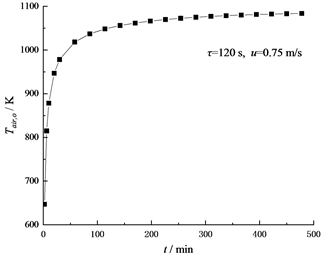Figure 6. Air outlet temperature at the end of exothermic periods

4. 预热过程中蓄热体的温度分布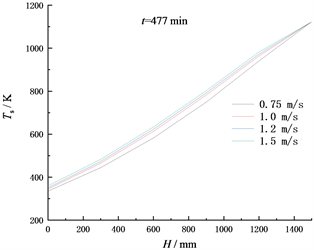(a) t = 477 min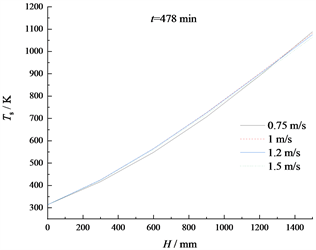(b) t = 478 min

Figure 7. Temperature distribution of honeycomb ceramics under different face velocity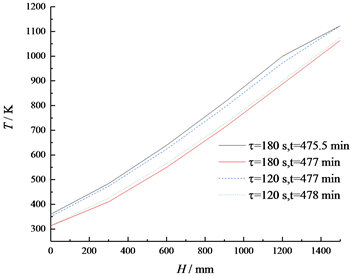Figure 8. Temperature distribution of honeycomb ceramics under different switching time

5. 堆积蜂窝陶瓷换热过程的温度效率

$\epsilon =\left({T}_{air,o}-{T}_{air,i}\right)/\left({T}_{gas,i}-{T}_{air,i}\right).$ (7)

Table 2. Temperature efficiency of honeycomb ceramic heat exchange process

6. 结论

1) 蓄热体预热过程中，高温烟气出口温度上升十分缓慢，上升幅度也很小；空气出口温度先急剧上升，然后升温速率迅速衰减。从蓄热体开始预热到换热过程达到稳定，所需时间均大于4小时。这表明蜂窝陶瓷蓄热体换热过程的热惰性很强，会使蓄热氧化炉对运行调节的响应速度比较慢，在蓄热氧化炉设计和运行过程中除了考虑静态特性，有必要通过数值计算进一步掌握蓄热氧化炉对气体流量、废气浓度等运行参数波动时的动态响应特性，为控制系统设计提供参考。

2) 蓄热体换热过程达到稳定以后，在相同的迎面流速下，换向时间越短，蓄热期烟气出口温度越低，放热期空气出口温度和温度效率越高；在相同的换向时间下，迎面流速越高，蓄热期烟气出口温度越高，放热期空气出口温度和温度效率越低；迎面流速对温度效率的影响比换向周期更大。

3) 在本文讨论的蓄热氧化炉常用设计参数范围内，选取迎面流速为0.75 m/s，换向时间为60 s时，在放热期末温度效率仍能达到95.15%，满足温度效率不低于95%的要求。在蓄热氧化炉设计时，应结合废气中挥发性有机物的浓度和燃点，合理选取通过蓄热体换热需要达到的温度效率和废气预热温度。废气中挥发性有机物的燃点越低，浓度和热值越高，设计选取的温度效率和废气预热温度就可以越低。在达到所需温度效率的前提下，选用较高的迎面流速，可以降低蓄热氧化炉中蓄热体的填充量、蓄热氧化炉体积和制造成本。当然，选用较高流速也会增加炉内流动阻力和运行成本，在工程设计中需要综合考虑设备投资和运行成本作进一步的技术经济分析。通过数值模拟获得的结果，可为蓄热氧化炉的热工设计提供有益的参考。

NOTES

*通讯作者。

  王波, 马睿, 薛国程, 等. 工业有机废气热氧化技术研究进展[J]. 化工进展, 2017, 36(11): 4232-4242.  王波, 商庆垄, 刘梦辉, 孙成喜, 王卓, 蔡晓锋. 蓄热氧化炉中蜂窝陶瓷损坏机理及改进措施[J]. 中国陶瓷, 2020, 56(2): 20-26.  韩东太, 荐蓓蓓. 蓄热体余热回收换热器强化传热实验研究[J]. 燃烧科学与技术, 2014, 20(1): 70-76.  欧俭平, 蒋绍坚, 萧泽强. 蜂窝型蓄热体传热过程热工特性的数值研究[J]. 耐火材料, 2003(6): 348-351.  刘永启, 牟宝杰, 郑斌, 刘瑞祥, 高振强. 莫来石蜂窝陶瓷的阻力特性[J]. 陶瓷学报, 2012, 33(2): 162-166.  郑志伟, 仇性启, 祁风雷, 等. 蜂窝陶瓷蓄热体传热和阻力特性实验研究[J]. 石油化工设备, 2013, 42(1): 9-13.  张振兴, 刘永启, 高振强, 等. 陶瓷蓄热体的流动与传热特性模拟研究[J]. 内燃机与动力装置, 2010(2): 22-26.  张振兴. 基于均匀多孔介质模型的氧化床阻力特性数值研究[D]: [硕士学位论文]. 淄博: 山东理工大学, 2010.  You, Y.H., et al. (2016) A Three-Dimensional Numerical Model of Unsteady Flow and Heat Transfer in Ceramic Honeycomb Regenerator. Applied Thermal Engineering, 108, 1243-1250. https://doi.org/10.1016/j.applthermaleng.2016.08.035  Coutinho, J.E.A. and De Lemos, M.J.S. (2012) Laminar Flow with Combustion in Inert Porous Media. International Communications in Heat & Mass Transfer, 39, 896-903. https://doi.org/10.1016/j.icheatmasstransfer.2012.06.002  李伟, 祁海鹰, 由长福, 等. 蜂巢蓄热体传热性能的数值研究[J]. 工程热物理学报, 2001, 22(5): 657-660.  吕志超, 周丽雯, 刘坤, 马光宇, 李卫东, 刘常鹏. 蜂窝陶瓷蓄热体传热及气体流动特性的数值模拟[J]. 冶金能源, 2017, 36(S2): 43-45.  王波, 刘梦辉, 商庆垄, 孙成喜. 蜂窝陶瓷蓄热体阻力特性的数值模拟[J]. 热能动力工程, 2020, 35(7): 152-158.  钟水库, 马宪国, 赵无非, 眭向荣. 蜂窝陶瓷蓄热体换热器热性能的实验分析[J]. 上海理工大学学报, 2004(4): 333-335.  王娟, 黄碧纯, 赵建国, 吴军良, 付名利, 叶代启. 基于Fluent的甲苯蓄热燃烧过程的数值模拟[J]. 环境工程学报, 2011, 5(6): 1341-1346.